﻿ 细粒含量对饱和砂类土小应变剪切模量的影响
«上一篇文章快速检索 高级检索

 哈尔滨工程大学学报2019, Vol. 40Issue (7): 1297-1303  DOI: 10.11990/jheu.2018050670

### 引用本文WU Qi, LI Xiaoxue, YANG Wenbao, et al. Influence of the fines content on the small-strain shear modulus characteristics of saturated sandy soils[J]. Journal of Harbin Engineering University, 2019, 40(7), 1297-1303. DOI: 10.11990/jheu.201805067.### 文章历史

1. 南京工业大学 岩土工程研究所, 江苏 南京 210009;
2. 江苏省土木工程防震技术研究中心, 江苏 南京 210009

Influence of the fines content on the small-strain shear modulus characteristics of saturated sandy soils
WU Qi 1, LI Xiaoxue 1, YANG Wenbao 1, ZHAO Kai 1, CHEN Guoxing 1,21. Institute of Geotechnical Engineering, Nanjing Technology University, Nanjing 210009, China;
2. Civil Engineering and Earthquake Disaster Prevention Center of Jiangsu Province, Nanjing 210009, China
Abstract: To investigate the influence of the fines content FC, the void ratio e, and the initial effective confining pressure σ'3c on the small-strain shear modulus Gmax of saturated sandy soils, a series of bender element tests was conducted on saturated sandy soils with various FC, e, and σ'3c. The test results show that, with the same σ'3c and FC, Gmax decreases with the increase of e, and with the same e and FC, Gmax increases with the increase of σ'3c. Moreover, when FC is the same, the decrease rate of Gmax remains unchanged with the increase of e, whereas when σ'3c is the same, FC has a strong influence on the decrease rate of Gmax with the increase of e. The analysis shows that, when FC is given, Gmax can be estimated well using the Hardin model. However, with the increase of FC, parameter A of the Hardin model decreases gradually. The modified Hardin model based on the equivalent skeleton void ratio can better predict Gmax of sandy soils with different FC, e, and σ'3c. Moreover, the error of the predicted Gmax is less than 10%.
Keywords: sandy soils    small-strain shear modulus    fines content    void ratio    equivalent skeleton void ratio    modified Hardin model

1 弯曲元试验 1.1 测试设备及测试方法

 ${V_{\rm{s}}} = d/t$ (1)

1.2 试验砂类土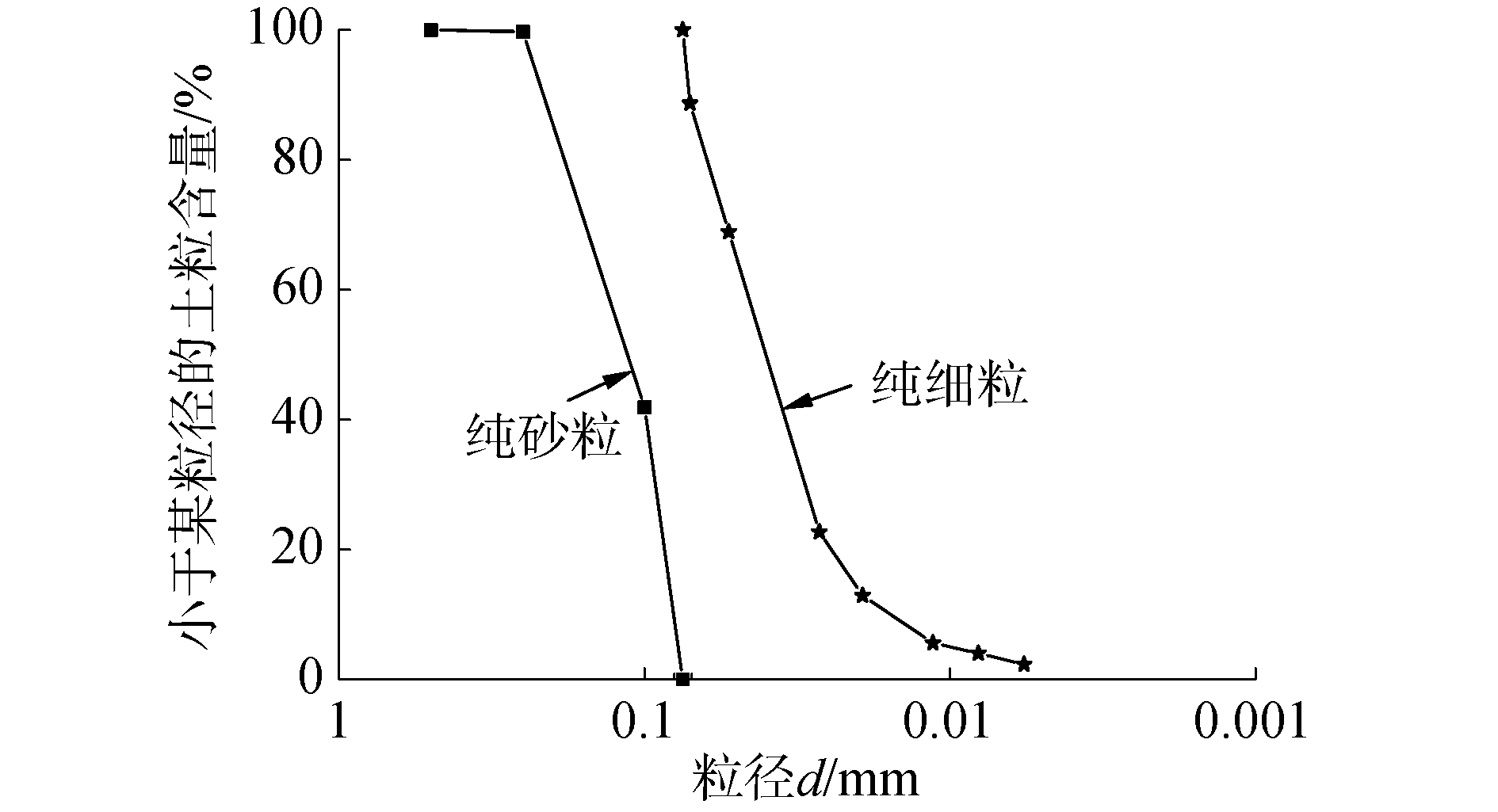Download: 图 1 砂类土的砂粒和细粒的级配曲线 Fig. 1 Grain size distributions of sand grains and fine grains for sandy soils
1.3 试样制备、饱和与固结

1.4 试验方案表 1 FC不同的砂类土的最大、最小孔隙比及比重 Table 1 Maximum, minimum void ratio and specific gravity of sandy soils with various FC表 2 砂-粉混合料的弯曲元试验工况 Table 2 Schemes of bender element tests for sand-silt mixtures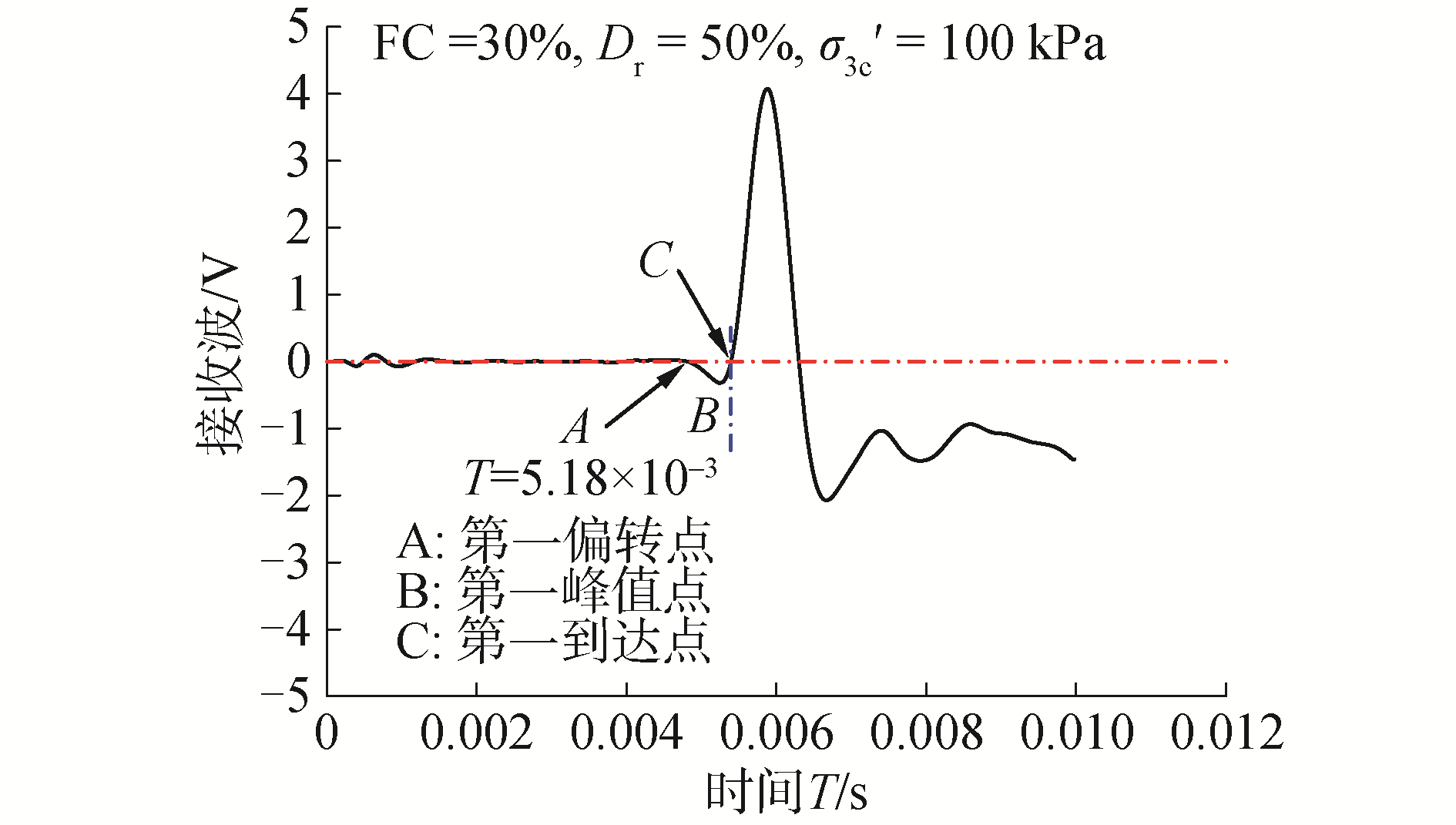Download: 图 2 典型的弯曲元接收端时程曲线 Fig. 2 Typical time history of output signal from bender element tests
2 试验结果与分析 2.1 砂类土Gmax的影响因素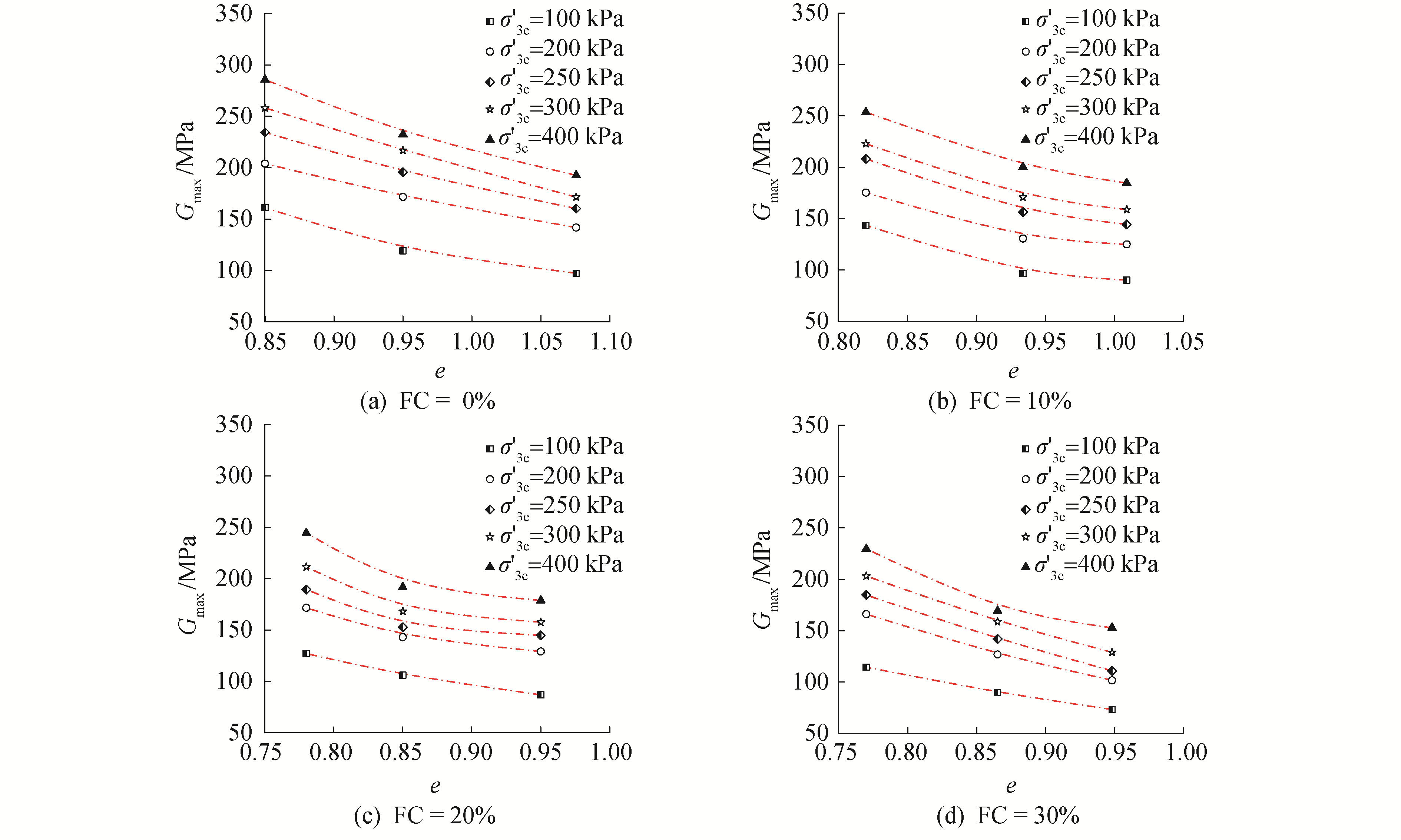Download: 图 3 Gmax与e的关系曲线 Fig. 3 The relationship between Gmax and e

 $F(e) = \frac{{{{(c - e)}^2}}}{{1 + e}}$ (2)

 ${G_{\max }} = A{P_{\rm{a}}}F(e){\left( {\frac{{\sigma _{3{\rm{c}}}^\prime }}{{{P_{\rm{a}}}}}} \right)^n}$ (3)

 ${G_{\max }} = A\frac{{{{(c - e)}^2}}}{{1 + e}}{\left( {\frac{{\sigma _{3{\rm{c}}}^\prime }}{{{P_{\rm{a}}}}}} \right)^{0.5}}$ (4)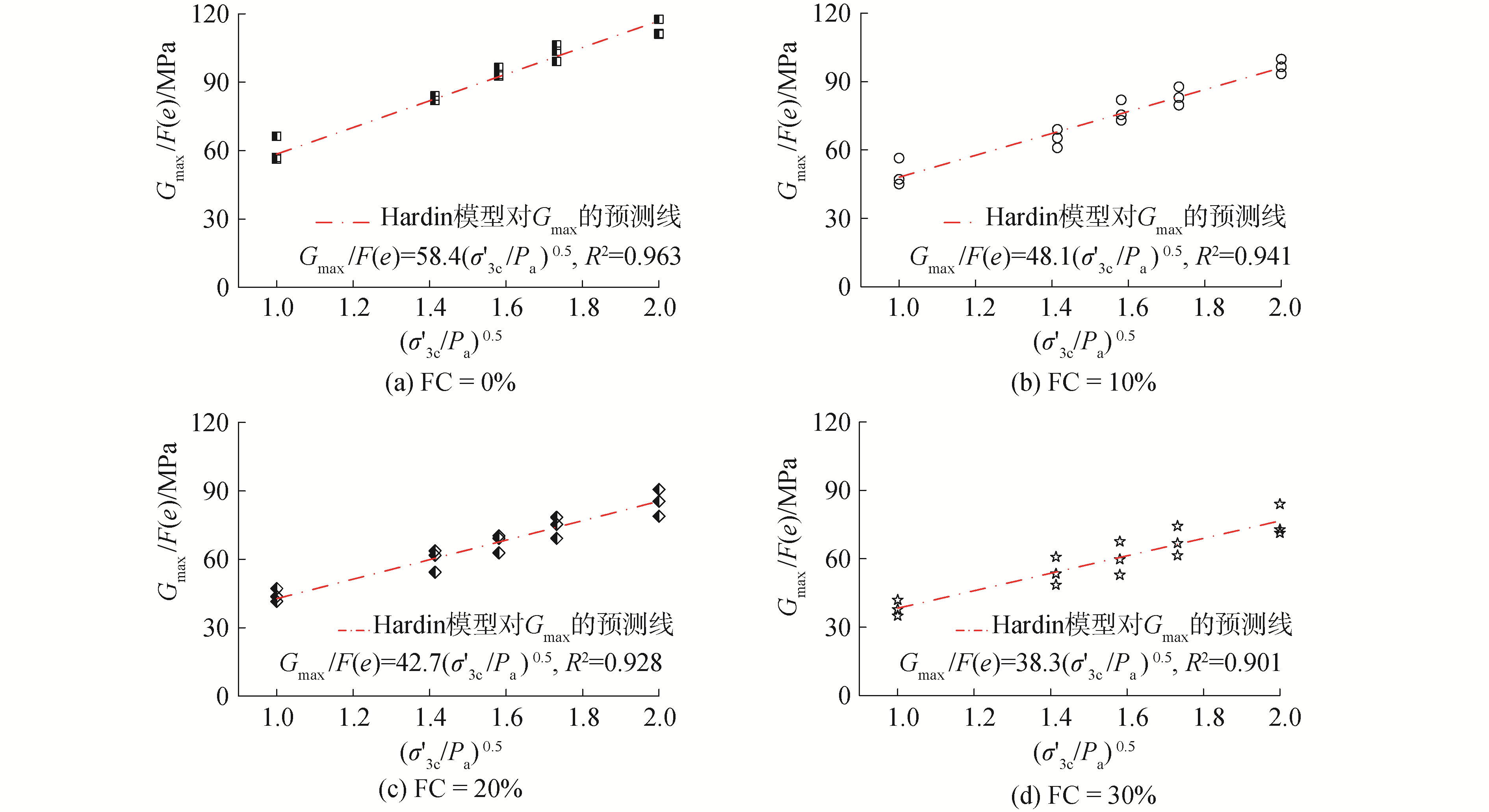Download: 图 4 FC不同的砂类土的Gmax/F(e)与(σ′3c/Pa)0.5的关系曲线 Fig. 4 The relationship between Gmax/F(e) and (σ′3c/Pa)0.5 for sandy soils with different FC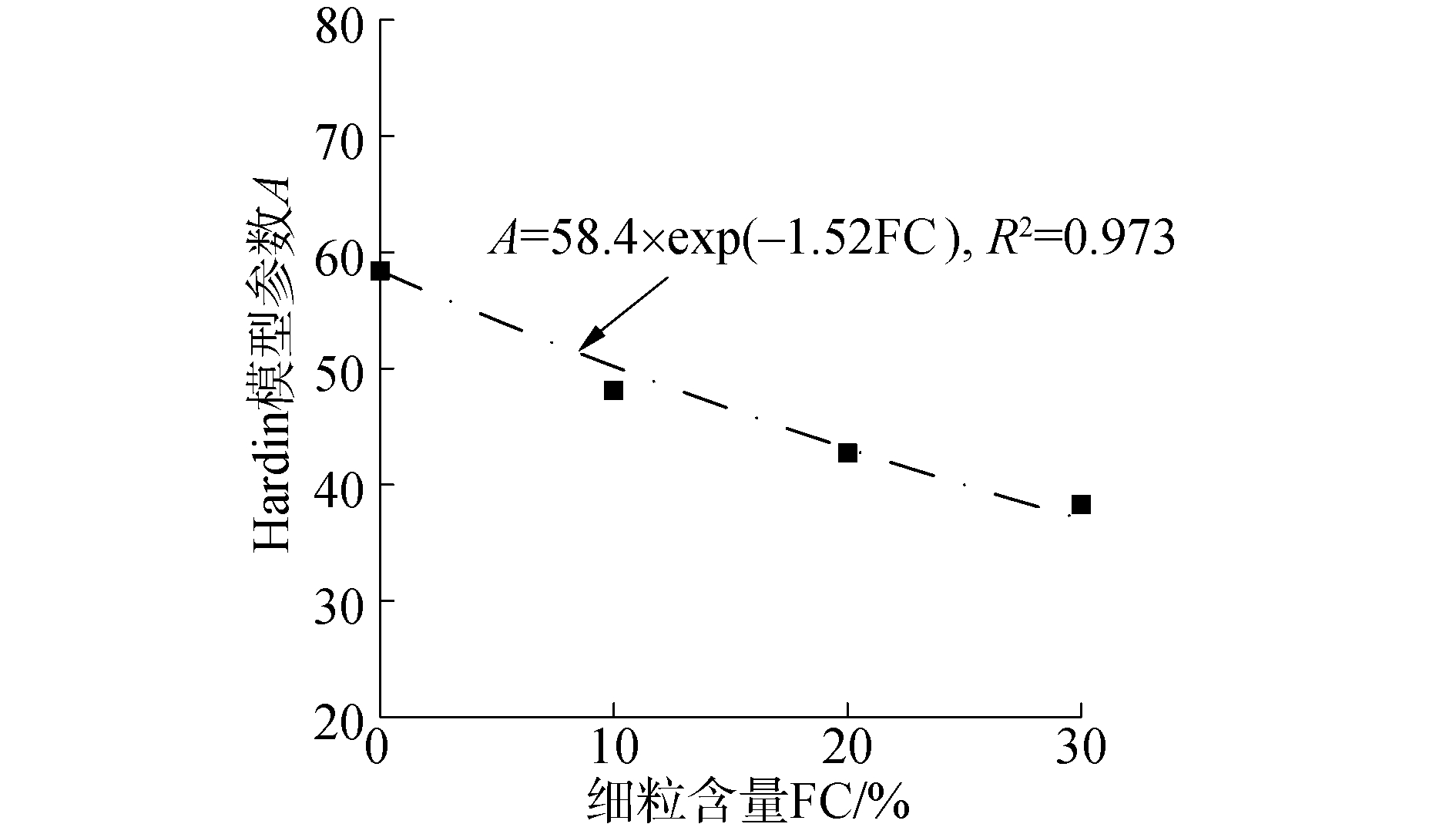Download: 图 5 Hardin模型参数A与FC的关系 Fig. 5 The relationship between A of Hardin model and FC for sandy soils
 $A({\rm{FC}}) = {A_0}\exp (nFC)$ (5)

2.2 修正Hardin模型对Gmax的评价

Thevanayagam等细颗粒的增加会明显改变砂类土的骨架颗粒组成，从而改变颗粒接触状态，当FC较小时，砂类土的颗粒处于“细粒填充砂粒”状态，砂粒组成土体骨架，其力学特性主要由砂粒组构决定。而当FC较大时，砂类土的颗粒处于“砂粒悬浮于细粒”状态，细粒组成土体骨架，此时力学特性主要由细粒组构决定。因此，对于不同颗粒接触状态的砂类土，必然存在着一个阈值细粒含量FCth(threshold fines content)以区分“细粒填充砂粒”与“砂粒悬浮于细粒”2种完全不同的颗粒接触状态。Rahman等基于9种砂类土的力学特性试验结果建立了预测FCth的经验公式：

 ${\rm{F}}{{\rm{C}}_{{\rm{th}}}} = 0.40 \times \left( {\frac{1}{{1 + \exp (0.50 - 0.13\chi )}} + \frac{1}{\chi }} \right)$ (6)

 $e_{{\rm{sk}}}^* = \frac{{e + (1 - b){\rm{FC}}}}{{1 - (1 - b){\rm{FC}}}}$ (7)

 $b = \left[ {1 - \exp \left( { - \frac{{0.3}}{k}} \right)} \right]{\left( {r \times \frac{{{\rm{FC}}}}{{{\rm{F}}{{\rm{C}}_{{\rm{th}}}}}}} \right)^r}$ (8)

 $F\left( {e_{{\rm{sk}}}^*} \right) = {\left( {c - e_{{\rm{sk}}}^*} \right)^2}/\left( {1 + e_{{\rm{sk}}}^*} \right)$ (9)

 ${G_{\max }} = AF\left( {e_{{\rm{sk}}}^*} \right){\left( {\sigma _{3{\rm{c}}}^\prime /{P_{\rm{a}}}} \right)^{0.5}}$ (10)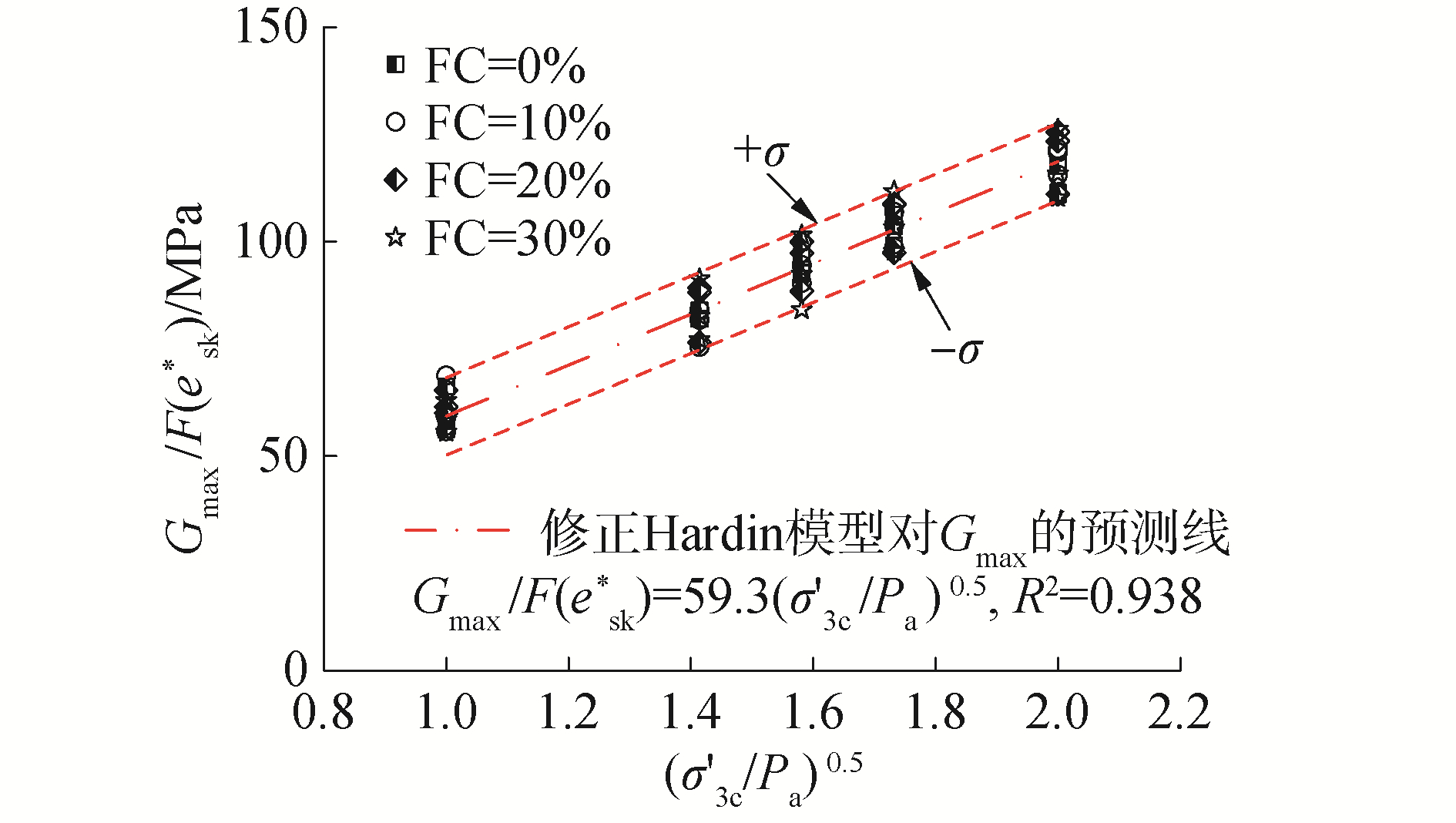Download: 图 6 FC不同的砂类土的Gmax/F(esk*)与(σ′3c/Pa)0.5的关系曲线 Fig. 6 The relationship between Gmax/F(esk*) and (σ′3c/Pa)0.5 for sandy soils with different FC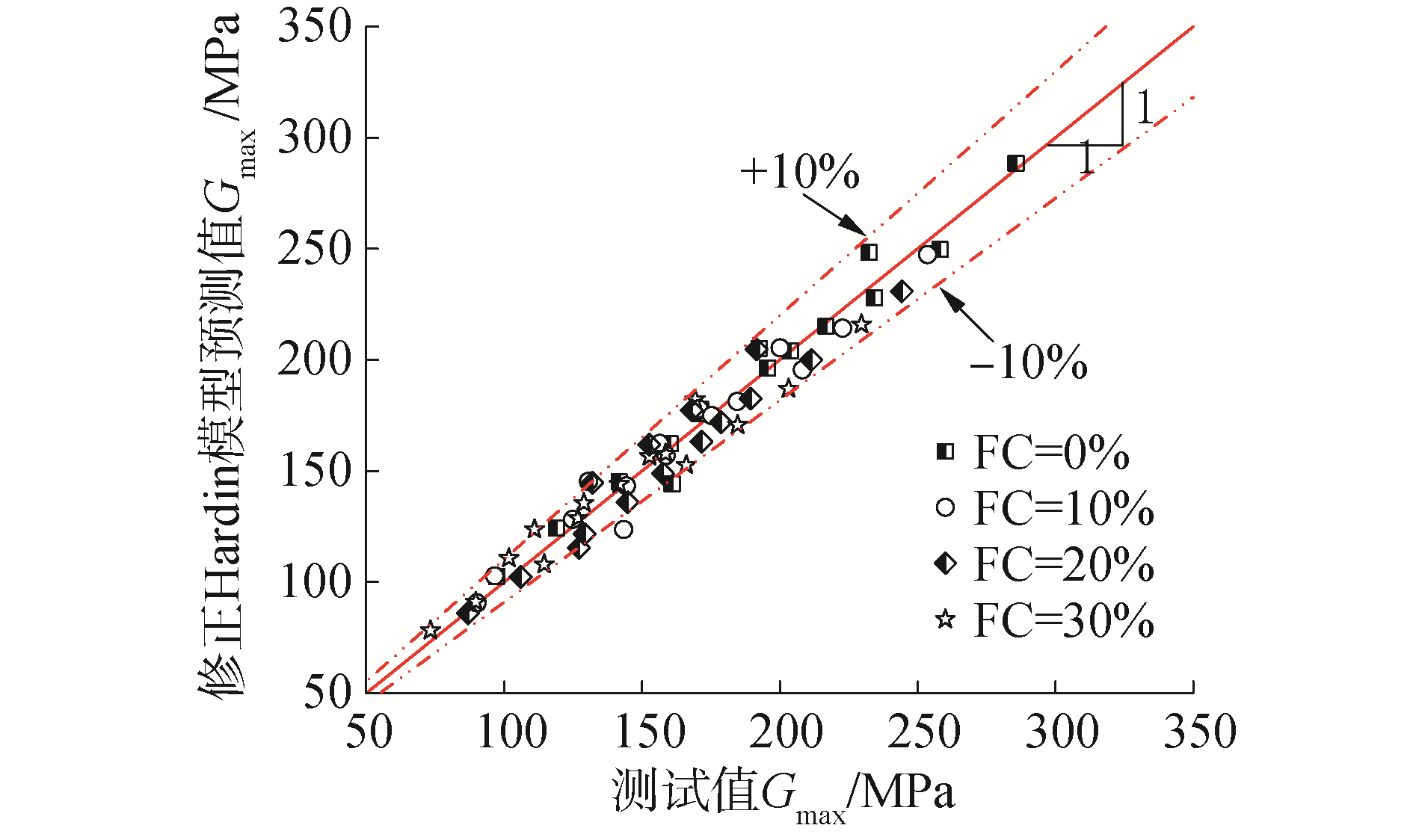Download: 图 7 Gmax的修正Hardin模型预测值与测试值的比较 Fig. 7 Comparison of the measured values and the predicted values using the modified Hardin model for the Gmax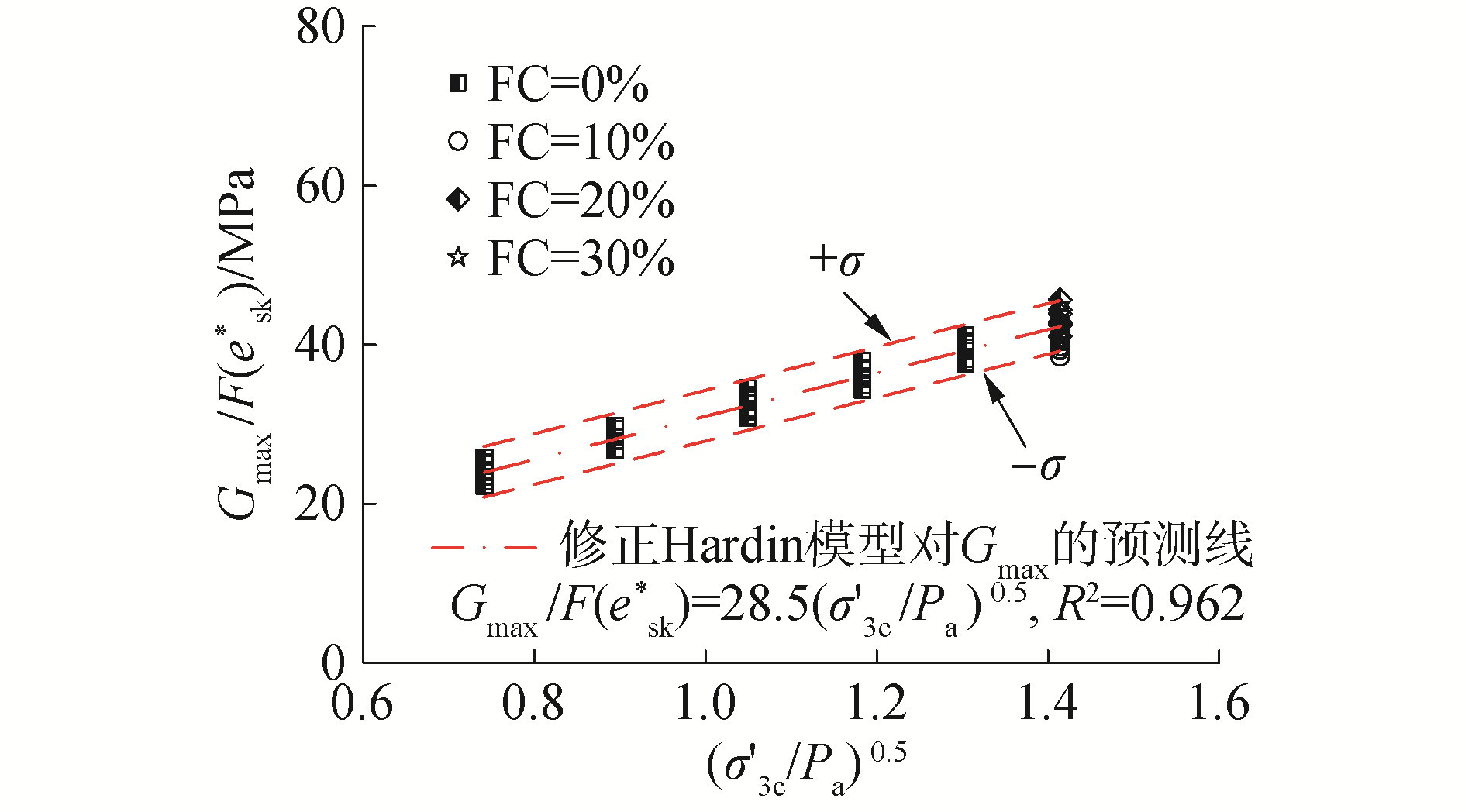Download: 图 8 修正Hardin模型对文献所述砂类土Gmax的评价 Fig. 8 Gmax evaluation for sandy soils in the literature  using the modified Hardin model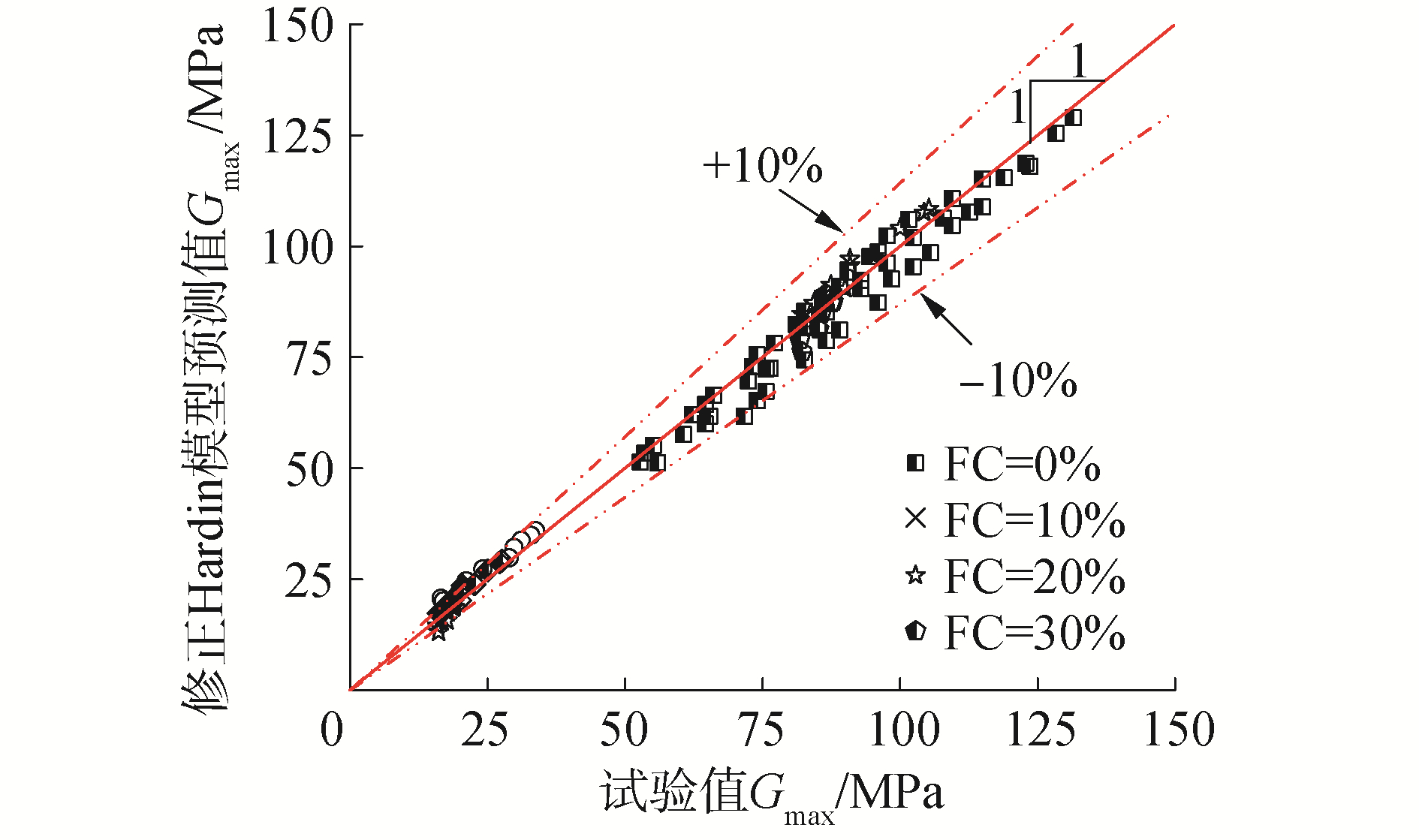Download: 图 9 修正Hardin模型对文献所述砂类土Gmax预测值与测试值的比较 Fig. 9 Comparison of the measured values and the predicted values using the modified Hardin model for the Gmax of sandy soil in the literature 
3 结论

1) 当其他条件相同时，Gmaxe的增大而减小；Gmaxσ3c的增大而增大，且σ3cGmaxe增大而减小的速率没有明显影响，而FC对Gmaxe增大而减小的速率有显著影响。

2) 对于特定FC，Hardin模型对砂类土的Gmax有良好的预测效果，但拟合参数A随FC的增大呈指数函数形式减小，这表明，随FC的增大，砂类土的颗粒接触面积减小，使得归准化Gmax/F(e)逐渐降低。Hardin模型无法统一描述FC对砂类土Gmax的影响。

3) 综合考虑砂1类土固结条件、密实状态及颗粒接触状态的修正Hardin模型能较好的预测FC、σ3ce不同的砂类土的Gmax，且其预测误差小于10%。此外，该修正模型可以适用于不同砂类土。

  YANG J, YAN X R. Site response to multi-directional earthquake loading:a practical procedure[J]. Soil dynamics and earthquake engineering, 2009, 29(4): 710-721. (0)  CLAYTON C R I. Stiffness at small strain:research and practice[J]. Géotechnique, 2011, 61(1): 5-37. DOI:10.1680/geot.2011.61.1.5 (0)  HARDIN B O, BLACK W L. Sand stiffness under various triaxial stresses[J]. Journal of soil mechanics & foundations division, 1966, 92(SM2): 27-42. (0)  GOUDARZY M, RAHEMI N, RAHMAN M M, et al. Predicting the maximum shear modulus of sands containing nonplastic fines[J]. Journal of geotechnical and geoenvironmental engineering, 2017, 143(9): 06017013. DOI:10.1061/(ASCE)GT.1943-5606.0001760 (0)  范明桥, 藤延京. GB/T 50145-2007, 土的工程分类标准[S].北京: 中国计划出版社, 2008. FAN Mingqiao, TENG Yanjing. GB/T 50145-2007, Standard for engineering classification of soil[S]. Beijing: China Planning Press, 2008. (0)  HSIAO D H, PHAN V T A, HSIEH Y T, et al. Engineering behavior and correlated parameters from obtained results of sand-silt mixtures[J]. Soil dynamics and earthquake engineering, 2015, 77: 137-151. DOI:10.1016/j.soildyn.2015.05.005 (0)  IWASAKI T, TATSUOKA F. Effects of grain size and grading on dynamic shear moduli of sands[J]. Soils and foundations, 1977, 17(3): 19-35. DOI:10.3208/sandf1972.17.3_19 (0)  HUANG Yaotao, HUANG Anbin, KUO Yuchen, et al. A laboratory study on the undrained strength of a silty sand from Central Western Taiwan[J]. Soil dynamics and earthquake engineering, 2004, 24(9/10): 733-743. (0)  OKA L G, DEWOOLKAR M, OLSON S M. Comparing laboratory-based liquefaction resistance of a sand with non-plastic fines with shear wave velocity-based field case histories[J]. Soil dynamics and earthquake engineering, 2018, 113: 162-173. DOI:10.1016/j.soildyn.2018.05.028 (0)  CARRARO J A H, PREZZI M, SALGADO R. Shear strength and stiffness of sands containing plastic or nonplastic fines[J]. Journal of geotechnical and geoenvironmental engineering, 2009, 135(9): 1167-1178. DOI:10.1061/(ASCE)1090-0241(2009)135:9(1167) (0)  SALGADO R, BANDINI P, KARIM A. Shear strength and stiffness of silty sand[J]. Journal of geotechnical and geoenvironmental engineering, 2000, 126(5): 451-462. DOI:10.1061/(ASCE)1090-0241(2000)126:5(451) (0)  CHOO H, BURNS S E. Shear wave velocity of granular mixtures of silica particles as a function of finer fraction, size ratios and void ratios[J]. Granular matter, 2015, 17(5): 567-578. DOI:10.1007/s10035-015-0580-2 (0)  吴琪, 杨文保, 朱雨萌, 等. 砂-粉混合料小应变剪切模量弯曲元试验研究[J]. 东南大学学报(自然科学版), 2018, 48(6): 1059-1067. WU Qi, YANG Wenbao, ZHU Yumeng, et al. Experimental study on small-strain shear modulus of sand-silt mixtures by bender element testing[J]. Journal of Southeast University (natural science edition), 2018, 48(6): 1059-1067. (0)  BRIGNOLI E G M, GOTTI M, STOKOE K H. Measurement of shear waves in laboratory specimens by means of piezoelectric transducers[J]. Geotechnical testing journal, 1996, 19(4): 384-397. DOI:10.1520/GTJ10716J (0)  陈云敏, 周燕国, 黄博. 利用弯曲元测试砂土剪切模量的国际平行试验[J]. 岩土工程学报, 2006, 28(7): 874-880. CHEN Yunmin, ZHOU Yanguo, HUANG Bo. International parallel test on the measurement of shear modulus of sand using bender elements[J]. Chinese journal of geotechnical engineering, 2006, 28(7): 874-880. DOI:10.3321/j.issn:1000-4548.2006.07.013 (0)  南京水利科学研究院. SL 237-1999, 土工试验规程[S].北京: 中国水利水电出版社, 1999. Nanjing Hydraulic Research Institute. SL 237-1999, Specification of soil test[S]. Beijing: China Water Power Press, 1999. (0)  ISHIHARA K. Liquefaction and flow failure during earthquakes[J]. Géotechnique, 1993, 43(3): 351-451. DOI:10.1680/geot.1993.43.3.351 (0)  YANG J, LIU X, RAHMAN M M, et al. Shear wave velocity and stiffness of sand:the role of non-plastic fines[J]. Géotechnique, 2018, 68(10): 931-934. DOI:10.1680/jgeot.16.D.006 (0)  RAHMAN M M, LO S R, GNANENDRAN C T. On equivalent granular void ratio and steady state behaviour of loose sand with fines[J]. Canadian geotechnical journal, 2008, 45(10): 1439-1456. DOI:10.1139/T08-064 (0)  THEVANAYAGAM S, SHENTHAN T, MOHAN S, et al. Undrained fragility of clean sands, silty sands, and sandy silts[J]. Journal of geotechnical and geoenvironmental engineering, 2002, 128(10): 849-859. DOI:10.1061/(ASCE)1090-0241(2002)128:10(849) (0)  MOHAMMADI A, QADIMI A. A simple critical state approach to predicting the cyclic and monotonic response of sands with different fines contents using the equivalent intergranular void ratio[J]. Acta geotechnica, 2015, 10(5): 587-606. DOI:10.1007/s11440-014-0318-z (0)  POLITO C P, MARTIN Ⅱ J R. Effects of nonplastic fines on the liquefaction resistance of sands[J]. Journal of geotechnical and geoenvironmental engineering, 2001, 127(5): 408-415. DOI:10.1061/(ASCE)1090-0241(2001)127:5(408) (0)  吴琪, 陈国兴, 朱雨萌, 等. 基于等效骨架孔隙比指标的饱和砂类土抗液化强度评价[J]. 岩土工程学报, 2018, 40(10): 1912-1922. WU Qi, CHEN Guoxing, ZHU Yumeng, et al. Evaluating liquefaction resistance of saturated sandy soils based on equivalent skeleton void ratio[J]. Chinese journal of geotechnical engineering, 2018, 40(10): 1912-1922. (0)  GOUDARZY M, RAHMAN M, KÖNIG D, et al. Influence of non-plastic fines content on maximum shear modulus of granular materials[J]. Soils and foundations, 2016, 56(6): 973-983. DOI:10.1016/j.sandf.2016.11.003 (0)